Courses

# Effect Of Addition Of Strong/Weak Acids And Bases - Ionic Equilibrium Chemistry Notes | EduRev

## Physical Chemistry

Created by: Asf Institute

## Chemistry : Effect Of Addition Of Strong/Weak Acids And Bases - Ionic Equilibrium Chemistry Notes | EduRev

The document Effect Of Addition Of Strong/Weak Acids And Bases - Ionic Equilibrium Chemistry Notes | EduRev is a part of the Chemistry Course Physical Chemistry.
All you need of Chemistry at this link: Chemistry

Effect of addition of strong electrolyte in weak electrolyte:

• Adding strong electrolyte which is non reacting

• Adding strong electrolyte which is reacting

• Adding strong electrolyte which is having common ion

Case I: Adding  non reacting electrolyte [CH3CooH + NaCl]

(0.1 M NaCl, volume V)+(0.1MCH3CooH, Volume V)

Now, concentration of

Use 0.05 for further calculation not 0.1, because we did mixing which leads to changes in concentration.

Case II: Adding strong electrolyte (reacting) [CH3OOH + AgNO3]

CH3COOH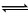CH3COO–    + H+

Let at eq.              10                                 5                5

New eq.               10-x                              4+x             5+x

AgNO3                    →          Ag+ + NO3

1                                           -           -

–                                           1          1

CH3COO + Ag+        →        white ppt.

Kα =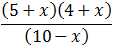III.  Adding strong electrolyte having common ion[ CH3COOH + HCl ]

CH3COOHCH3COO + H+

C1                                    –               –

Eq.       C1 – C1α                      C1α      C1α

Strong electrolyte                    HCl →  H+  + Cl

C2          –       –

–            C2    C2

Here added species HCl dissociated to give common ion H+.

Because of excess concentration of H+, equilibrium shift, to backward direction. Thus,

CH3COOHCH3COO + H+

Equation                           C1 – C1α1                     C1α1              C1α1

Here    α1< α

Because equilibrium is shifted to backward direction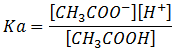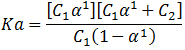Considering α1<<< 1

C1α1<<< C2

Hence C1α1 + C2≈C2

So, Kα =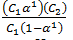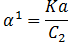Note:

Here C2 is the concentration of common ion Not the concentration of acid added.

Example: Calculate the degree of dissociation and pH of the solution

1. If 0.1 m aqueous solution of ammonia is present.

2. If 0.1 m aqueous solution of ammonia is present in presence of 0.05 m Ca(OH)2, Kb = 105

NH4OHNH4+ + OH

C - Cα                        Cα       Cα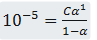α <<< 1

α = 102

The value of a is less than 0.05. hence neglection of a is correct.

[OH] = Ca = 10–1 × 10–2 = 10–3

POH = – log [OH] = –log (10–3)]

POH = 3

pH = 14–3 = 11

pH = 11

b)    NH4OHNH4+ + OH

C - Cα1                        Cα1       1

Cα(OH)2      →              Cα2+ + 2 OH

0.05                              -                -

-                                   0.05         0.1

Kb =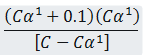After neglection, 105 = (Cα1 + 0.1) 1

105 = 0.1 × 1

α1 = 104

[OH] = Cα1 + C2 = 105 + 101 = 101         (105 is neglected as compared to 101)

[OH] = 0.1

[H+] [OH] = 1014

[H+] =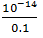pH = 13

Addition of weak electrolyte on weak electrolyte

HH+ + A             Kα1   =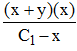[C1 – x ≈ C1]

C1                    –          –                      Kα1C1 = (x+y)(x)

C1 – x              x+y      x

HBH++ B                            Kα2  =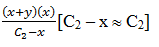C2                    –        –

C2 – x              x+y      y                       Kα2 C = (x+y) (x)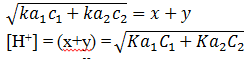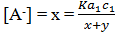Remember, this Formula is after neglection. We have considered that x and y are neglected as compared to C1

Example: Acetic acid with Kα = 105 is present in an aqueous solution. Calculate the pH of solution if

a)         When only 0.1M acetic acid is present

b)         When only 0.1 Molar acetic acid is present along 0.05 m H2SO4.

c)         When 1L acetic acid (0.1 M) is mixed with 1L, 0.1 M HCl

Solution:

a)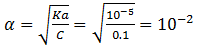CH3COOHCH3COO   + H2

C - Cα                         Cα               Cα

[H+] = Cα

= 101 x 102 = 103

pH = – log [H+] = 3

pH = 3

b)   H2SO4→ 2H+ + SO42

0.05            –          –

–               0.1      0.05

CH3COOHCH3COO   + H+

C - Cα1                        Cα1               Cα1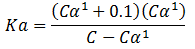Ka = 0.1α1

α1 = 104

[H+] = Cα1 + 0.1 = 101 × 104 + 0.1

[H+] = 105 + 101

[H+] = 101 (we have neglected terms that are less than ten times)

pH = 1

c)         Here, first you have to calculate the concentration of species after mixing

Concentration of CH3COOH =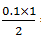= 0.05 M

Concentration of HCl = 0.05 M

CH3COOHCH3COO + H+

C - Cα1                        Cα1              Cα1

HCl     →        H+    +   Cl

0.05                 –              –

-                       0.05     0.05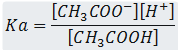After considering neglection.

Kα =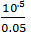= 2 X 10-4

[H+] = 0.05 + Cα1

[H+] = 0.05

pH = - log (0.05)

pH = 1.3

Example: 0.1 M NaOH, 200 ml is mixed with 0.1m, 100 ml Ca(OH)2. Find the pH of the solution.

Solution: 0.1 M NaOH, 200 ml + 0.1 M, 100 ml Ca(OH)2

For NaOH = n OH = 0.1 × 200 = 20 mmol

Total no. of milimoles of OH = 40 mmol

[OH] =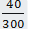POH =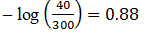pH = 13.12

Effect of addition of strong Acid on strong base:

When a strong acid is mixed with strong base,

An acid – base Reaction takes place, until the limiting reagent is finished.

For ex: When 0.1M, 100 ml HCl is mixed with 0.1 M, 50 ml NaOH.

It’s obvious the fastest reaction i.e., the Acid – base reaction takes place firstly.

HCl + NaOH→NaCl + H2O

Milimoles        10          5

5          -                 5

(nH+) left = 5 milimoles

Example: 0.1 M, 100 ml HCl is mixed with 0.1M, 100 ml (Ca(OH)2. Find the pH of the solution.

Solution: As the question itself revealing. When an acid and base is mixed, Firstly acid - Base Reaction takes place.

2 HCl + Ca(OH)2→ CaCl2 + H2O

Milimoles of Ca(OH)2 = 0.1 × 100 = 10 mmol

2 HCl + Ca(OH)2→ CaCl2 + H2O

10            10

–               5                 5

Now we have only Ca(OH)2 in the solution. It will completely dissociate to give Ca2+ and 2OH

Ca(OH)2→ Ca2+ + 2OH

5                    -             -

-                   5         10

(OH) =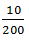= .05

pOH = 1.3

Hence pH = 12.7

Offer running on EduRev: Apply code STAYHOME200 to get INR 200 off on our premium plan EduRev Infinity!

## Physical Chemistry

77 videos|83 docs|32 tests

,

,

,

,

,

,

,

,

,

,

,

,

,

,

,

,

,

,

,

,

,

;